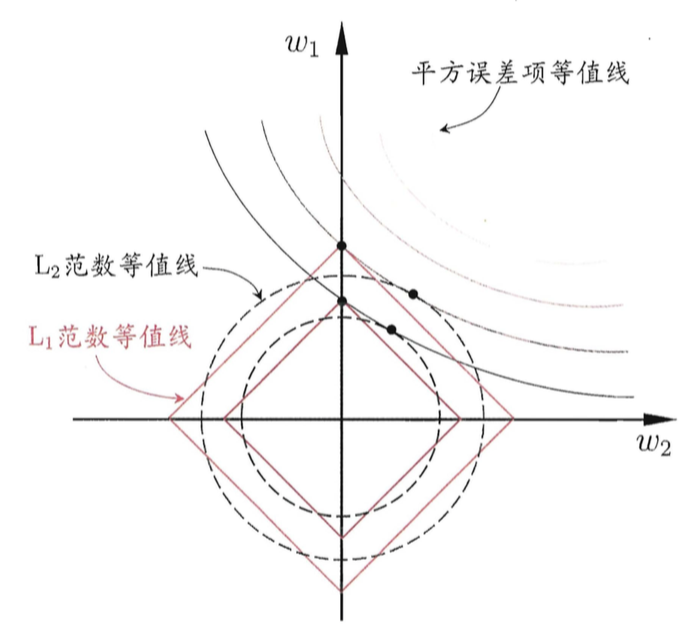# 一、损失函数与目标函数

$l(y, \hat y) = l(y, F(\vec x))$

$F^{*} = \mathop{\text{arg min}}_{F} E_{y,\,\vec x}\Bigl[l\bigl(y,\,F(\vec x)\bigr)\Bigr] = \mathop{\text{arg min}}_{F}L(F)$

$F^{*} = \mathop{\text{arg min}}_{F}\text{Obj}(F) = \mathop{\text{arg min}}_{F}L(F) + \gamma\Omega(F)$

# 二、范数与正则项

## 2.1 定义

• 非负性：$\lVert\vec x\rVert\geqslant 0$
• 齐次性：$\lVert c\cdot\vec x\rVert = \lvert c\rvert \cdot \lVert\vec x\rVert$
• 三角不等式：$\lVert \vec x + \vec y\rVert \leqslant \lVert\vec x\rVert + \lVert\vec y\rVert$

• $L_0$-范数：$\lVert\vec x\rVert_0 = \#(i)$$i$不等于0；
• $L_1$-范数：$\lVert\vec x\rVert_1 = \sum_{i = 1}^{d}\lvert x_i\rvert$
• $L_2$-范数：$\lVert\vec x\rVert_2 = \Bigl(\sum_{i = 1}^{d}x_i^2\Bigr)^{1/2}$
• $L_p$-范数：$\lVert\vec x\rVert_p = \Bigl(\sum_{i = 1}^{d}x_i^p\Bigr)^{1/p}$
• $L_\infty$-范数：$\lVert\vec x\rVert_\infty = \lim_{p\to+\infty}\Bigl(\sum_{i = 1}^{d}x_i^p\Bigr)^{1/p}$

## 2.2 L1和L2 范数的对比

### 2.2.1 鲁棒性： L1＞L2

$L_1$范数比$L_2$范数更鲁棒，原因相当明显：从定义中可以看到，$L_2$范数取平方值，因此它以指数方式增加异常值的影响；$L_1$范数只取绝对值，因此它会线性地考虑它们。

### 2.2.3 解的数量：L1多个，L2一个### 2.2.4 稀疏性：L1＞L2

• $L_1$-范数：$\lVert\vec w\rVert_1 = \sum_{i = 1}^{d}\lvert w_i\rvert$
• $L_2$-范数：$\lVert\vec w\rVert_2 = \Bigl(\sum_{i = 1}^{d}w_i^2\Bigr)^{1/2}$

$\frac{dL_1(w)}{dw} = sign(w)，\frac{dL_2(w)}{dw} = w$# 三、为什么正则化可以防止过拟合

$h_{\theta}(x) = \theta_0 + \theta_1x + \theta_2 x^2 …$

$J(\theta) = \frac{1}{2m} \sum_{i=1}^m (h_{\theta}(x^i) - y^i) ^ 2$

$\theta_j = \theta_j - \alpha \frac{\partial J(\theta)}{\partial \theta_j}$

$\frac {\partial J(\theta)}{\partial \theta_i} =\frac{1}{2m} \sum_{i=1}^m \frac{\partial(h_{\theta}(x^i) - y^i) ^ 2}{\partial \theta_j}= \frac{2}{2m} \sum_{i=1}^m (h_{\theta}(x^i) - y^i) \frac{\partial h_{\theta}(x^i)}{\partial \theta_j}$

$\frac{\partial h_{\theta}(x^i)}{\partial \theta_j} = \frac{\partial (\theta_0 x_0^i + \theta_1 x_1^i + \cdots+ \theta_j x_j^i + \cdots)}{\partial \theta_j} \ = \frac{\partial ( \theta_j x_j^i )}{\partial \theta_j} = x_j^i$

$\theta_j = \theta_j - \alpha \frac{1}{m} \sum_{i=1}^m {(h_{\theta}(x^i) - y^i)} x_j^i \tag 1$

（1）式是没有添加$L_2$正则化项的迭代公式，如果在原始代价函数之后添加$L_2$正则化，则迭代公式会变成下面的样子：
$\theta_j = \theta_j (1-\alpha\frac{\lambda}{m})- \alpha \frac{1}{m} \sum_{i=1}^m {(h_{\theta}(x^i) - y^i)} x_j^i \tag 2$

# 参考文献：